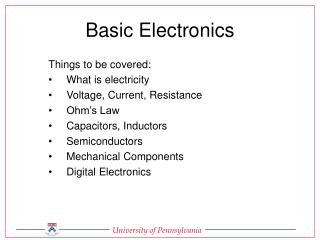# Basic Electronics - PowerPoint PPT PresentationDownload PresentationBasic Electronics

Basic Electronics
Download Presentation## Basic Electronics

- - - - - - - - - - - - - - - - - - - - - - - - - - - E N D - - - - - - - - - - - - - - - - - - - - - - - - - - -
##### Presentation Transcript

1. Basic Electronics Things to be covered: • What is electricity • Voltage, Current, Resistance • Ohm’s Law • Capacitors, Inductors • Semiconductors • Mechanical Components • Digital Electronics

2. What is Electricity • Everything is made of atoms • There are 118 elements, an atom is a single part of an element • Atom consists of electrons, protons, and neutrons

3. Electrons (- charge) are attracted to protons (+ charge), this holds the atom together • Some materials have strong attraction and refuse to loss electrons, these are called insulators (air, glass, rubber, most plastics) • Some materials have weak attractions and allow electrons to be lost, these are called conductors (copper, silver, gold, aluminum) • Electrons can bemade to move from one atom to another, this is called a current of electricity.

4. Surplus of electrons is called a negative charge (-). A shortage of electrons is called a positive charge (+). • A battery provides a surplus of electrons by chemical reaction. • By connecting a conductor from the positive terminal to negative terminal electrons will flow.

5. Voltage • A battery positive terminal (+) and a negative terminal (-). The difference in charge between each terminal is the potential energy the battery can provide. This is labeled in units of volts. Water Analogy

6. Voltage Sources:

7. Voltage is like differential pressure, • always measure between two points. • Measure voltage between two points • or across a component in a circuit. • When measuring DC voltage make • sure polarity of meter is correct, • positive (+) red, negative (-) black.

8. Ground

9. Exercise • Measure DC voltage from power supply using multimeter • Measure DC voltage from power supply using oscilloscope • Measure DC voltage from battery using multimeter • Measure AC voltage from wall outlet using a multimeter • Measure AC voltage from wall outlet using an oscilloscope Effective or Root Mean Square Voltage (Measured with multimeter) ERMS=0.707xEA E

10. Current • Uniform flow of electrons thru a circuit is called current. WILL USE CONVENTIONAL FLOW NOTATION ON ALL SCHEMATICS

11. To measure current, must break circuit and install meter in line. • Measurement is imperfect because of voltage drop created by meter.

12. Resistance • All materials have a resistance that is dependent on cross-sectional area, material type and temperature. • A resistor dissipates power in the form of heat

13. Various resistors types

14. When measuring resistance, remove component from the circuit.

15. Resistor Color Code

16. Exercise • Determine the resistance of various resistors of unknown value using the resistor color code • Using the multimeter, compare the specified resistance and measured resistance • Using the multimeter to examine the characteristics of various potentiometers

17. Ohm’s Law

18. Prototyping Board Example of how components are Inserted in the protoboard

19. Exercise • Calculate the total current and voltage drop across each resistor shown in Figure 1 • Build the circuit in Figure 1 on the prototype board • Measure the total circuit current and voltage drops across each resistor and compare • the calculated and measured values

20. Capacitance A capacitor is used to store charge for a short amount of time Capacitor Battery Unit = Farad Pico Farad - pF = 10-12F Micro Farad - uF = 10-6F

21. Capacitor Charging

22. Capacitor Discharge

23. Inductance